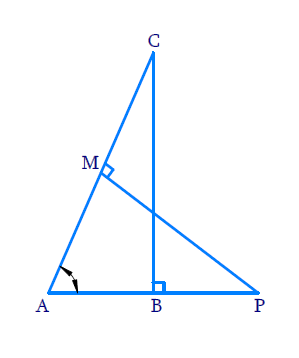In the verge of coronavirus pandemic, we are providing FREE access to our entire Online Curriculum to ensure Learning Doesn't STOP!

# Ex.6.3 Q9 Triangles Solution - NCERT Maths Class 10

Go back to  'Ex.6.3'

## Question

In Figure, $$ABC$$ and $$AMP$$ are two right triangles, right angled at $$B$$ and $$M$$ respectively.

Prove that:

(i) \begin{align}\Delta ABC \sim \Delta AMP\end{align}

(ii) \begin{align}\frac{CA}{PA}=\frac{BC}{MP}\end{align}

DiagramVideo Solution
Triangles
Ex 6.3 | Question 9

## Text Solution

(i) Reasoning:

If two angles of one triangle are respectively equal to two angles of another triangle, then the two triangles are similar.

This may be referred to as the $$AA$$ similarity criterion for two triangles.

Steps:

In $$\Delta {ABC}$$ and $$\Delta {AMP}$$

\begin{align} & \angle ABC=\angle AMP={{90}^{^\circ }} \\& \,\angle BAC\!=\!\angle MAP\\& \qquad ( \text{Common angle}) \\\\& \;\;\Delta ABC\sim\Delta AMP \\\end{align}

(ii) Reasoning:

As we know that the ratio of any two corresponding sides in two equiangular triangles is always the same

Steps:

In $$\Delta {ABC}$$  and  $$\Delta {AMP}$$

\begin{align}\frac{CA}{PA}&=\frac{BC}{MP}\\(\because \Delta ABC &\sim \Delta AMP)\end{align}

Learn from the best math teachers and top your exams

• Live one on one classroom and doubt clearing
• Practice worksheets in and after class for conceptual clarity
• Personalized curriculum to keep up with school